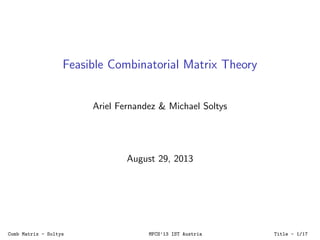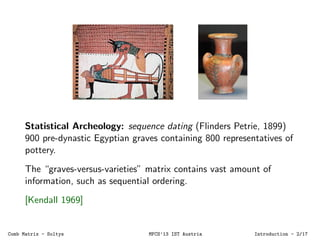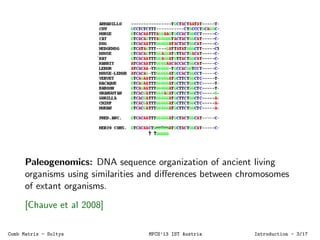Successfully reported this slideshow.

# Feasible Combinatorial Matrix Theory - MFCS2013×

1 of 21
1 of 21

# Feasible Combinatorial Matrix Theory - MFCS2013

### Feasible Combinatorial Matrix Theory - MFCS2013

1. 1. Feasible Combinatorial Matrix Theory Ariel Fernandez & Michael Soltys August 29, 2013 Comb Matrix - Soltys MFCS’13 IST Austria Title - 1/21
2. 2. Statistical Archeology: sequence dating (Flinders Petrie, 1899) 900 pre-dynastic Egyptian graves containing 800 representatives of pottery. The “graves-versus-varieties” matrix contains vast amount of information, such as sequential ordering. [Kendall 1969] Comb Matrix - Soltys MFCS’13 IST Austria Introduction - 2/21
3. 3. Paleogenomics: DNA sequence organization of ancient living organisms using similarities and diﬀerences between chromosomes of extant organisms. [Chauve et al 2008] Comb Matrix - Soltys MFCS’13 IST Austria Introduction - 3/21
4. 4. Consecutive-ones Property: C1P Consider a slight relaxation, (k, δ)-C1P: each row has at most k blocks of 1s and the gap between any two blocks is at most δ. So (1, 0)-C1P is C1P, and deciding if an A has (k, δ)-C1P is: polytime for (1, 0) NP-hard for every k ≥ 2, δ ≥ 1 except (2, 1) What about (2, 1)? [Patterson 2012] Comb Matrix - Soltys MFCS’13 IST Austria Introduction - 4/21
5. 5. K¨onig’s Min-Max 0 1 0 1 1 1 0 1 00 0 1 1 0 00 Comb Matrix - Soltys MFCS’13 IST Austria Introduction - 5/21
6. 6. Comb Matrix - Soltys MFCS’13 IST Austria Introduction - 6/21
7. 7. Problems Cover(A, α): ∀i, j ≤ r(A)(A(i, j) = 1 → α(1, i) = 1 ∨ α(2, j) = 1) MinCover(A, α): Cover(A, α) ∧ ∀α ≤ c(α)(Cover(A, α ) → Σα ≥ Σα) KMM(A, α, β): MinCover(A, α) ∧ MaxSelect(A, β) → Σα = Σβ a ΣB 1 formula. But classical proof is ΠB 2 — is there a ΣB 1 proof? Comb Matrix - Soltys MFCS’13 IST Austria Problem - 7/21
8. 8. Related Theorems: Menger’s: size of min cut equals max nr of disjoint s, t-paths (“Min-Cut Max-Flow”) Hall’s: ∀k ∈ [n] |Si1 ∪ . . . ∪ Sik | ≥ k, then there exists a System of Distinct Representatives. Dilworth’s: Min nr of chains needed to partition a poset equals size of max anti-chain of that poset. Can they all be shown equivalent to KMM with ΣB 0 proofs? Comb Matrix - Soltys MFCS’13 IST Austria Problem - 8/21
9. 9. ΣB 1 Proof of KMM Let lA be the min nr of lines necessary to cover A Let oA be the max selection of ones in A Comb Matrix - Soltys MFCS’13 IST Austria Main Proof - 9/21
10. 10. 0 1 0 1 1 1 0 1 00 0 1 1 0 00 lA=oA= 3 We want to show with ΣB 1 induction that oA = lA. Comb Matrix - Soltys MFCS’13 IST Austria Main Proof - 10/21
11. 11. LA uses ΣB 0 induction LA oA ≤ lA LA over Z is equivalent to VTC0 And oA ≤ lA follows more or less from the Pigeonhole Principle: if we can select oA 1s, no two on the same line, then we shall require at least lA lines to cover those 1s. Comb Matrix - Soltys MFCS’13 IST Austria Main Proof - 11/21
12. 12. ∃LA oA ≥ lA Showing oA ≥ lA is more diﬃcult; we use ΣB 1 induction. Lots of cases, but the interesting case is: 0 where we reduce the general case to the case of the blue matrix whose cover requires as many lines as rows. Comb Matrix - Soltys MFCS’13 IST Austria Main Proof - 12/21
13. 13. 00 00 00 0 0 0 0 0 0 Comb Matrix - Soltys MFCS’13 IST Austria Interesting Case - 13/21
14. 14. 10 00 00 0 0 0 0 0 0 Comb Matrix - Soltys MFCS’13 IST Austria Interesting Case - 14/21
15. 15. 0 0 00 00 0 0 0 0 0 01 0 0 0 1 0 0 0 0 0 Comb Matrix - Soltys MFCS’13 IST Austria Interesting Case - 15/21
16. 16. 1 0 00 00 0 0 0 0 0 01 0 0 0 1 0 0 0 0 0 Comb Matrix - Soltys MFCS’13 IST Austria Interesting Case - 16/21
17. 17. 1 0 00 00 0 0 0 0 0 01 0 0 0 1 0 0 0 0 0 Comb Matrix - Soltys MFCS’13 IST Austria Interesting Case - 17/21
18. 18. H 1 1 1 1 1 1 1 1 1 1 1 1 1 1 1 1 A B C D E FG Comb Matrix - Soltys MFCS’13 IST Austria Interesting Case - 18/21
19. 19. J 1 1 1 1 1 1 1 1 1 1 1 1 1 1 1 1 H 1 1 Comb Matrix - Soltys MFCS’13 IST Austria Interesting Case - 19/21
20. 20. ΣB 0 Proof of Equivalence For example, ΣB 0 proof of Menger → KMM yx Left graph has a matching of size k ⇐⇒ Right graph has k disjoint {x, y}-paths Left graph has a cover of size k ⇐⇒ Right graph has an {x, y}-cut of size k KMM → Meng complicated, [Aharoni 1983] Comb Matrix - Soltys MFCS’13 IST Austria Proof of equivalences - 20/21
21. 21. Some open problems Frankl’s Theorem: t a positive integer, and m ≤ n(2t −1) t ; if A is m × n, and its rows are distinct, then there exists a column that when deleted, the resulting matrix has at most 2t−1 − 1 pairs of equal rows. (Bondy’s Theorem when t = 1.) Complexity of decompositions: A = P1 + P2 + · · · + Pn + X; n boys and n girls, each boy introduced to exactly k girls and vice versa. Compute a pairing where each boy & girl has been previously introduced. Projective Geometry          1 1 1 0 0 0 0 1 0 0 1 1 0 0 1 0 0 0 0 1 1 0 1 0 1 0 1 0 0 1 0 0 1 0 1 0 0 1 1 0 0 1 0 0 1 0 1 1 0          Desargues Thm Comb Matrix - Soltys MFCS’13 IST Austria Conclusion - 21/21